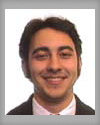#Wolfram Summer School

# Alumni# Roberto Martinez

Summer School

Class of 2003

## Bio

Roberto is a graduate research assistant and teaching fellow in Applied Physics in the Division of Engineering and Applied Sciences at Harvard University. Over the past five years, he has done research in such diverse areas as number theory and condensed matter physics to algebraic combinatorics and synthesis of organic semiconductors and superconductors. Specifically, he discovered an infinite class of transcendental numbers, co-discovered the largest base 7 prime in the world (30*7^80139+1, 67727 digits), synthesized a new liquid crystal, developed a globally bounded, nonlinear approximation/perturbation technique, developed a graph theoretic understanding of the Somos-4 sequence. At present, he is focused on quantum tunneling in nanostructures, topological field theory/knot theory and the axiomatization of physics, Hilbert’s sixth problem. In addition, he is writing a monograph on nested recursive functions, a continuation of his project at the NKS Summer School.

## Project: Regularity, Complexity and Discrete Chaos in Nested Recursive Functions

The mulitply recursive functions, for instance, with two terms at depth 2, f(n) = af(n – cf(n – 1)) + bf(n – df(n-2)), along with the initial conditions f(n) = 1 for n < 1 are shown to have four types of behavior; uniform, repetitive, nested and chaotic. These recursive functions are generalizations of Hofstadter’s function. In this work, certain classes were surveyed and the behaviors for the simplest cases are classified.

Particular attention is paid to regularities in their long-term behavior, or by modeling the long-term behavior. Evidence is given for Roberto’s conjecture that the growth of f[n_]:= f[n- a f[n-1]] + f[n- b f[n-2]] is asymptotic to the line n/(a + b), implying an overall regularity of the function.

Certain cases are shown to be computationally reducible, by giving formulas. With a single depth two term, f[n_]:=f[n]=a f[n-b f[n-c]], the values not equal to a are given by a^Floor[2(n+ab-1)/ab] under the assumption that ab = 0 mod 2c and b > c – 1.

## Wolfram Summer School | Champaign, IL, USA | July 3 29–July 22, 2022

APPLY NOW
• 21st Annual Wolfram Summer School
• Bentley University, Waltham, MA, USA
• June 25–July 15, 2023
• Apply now